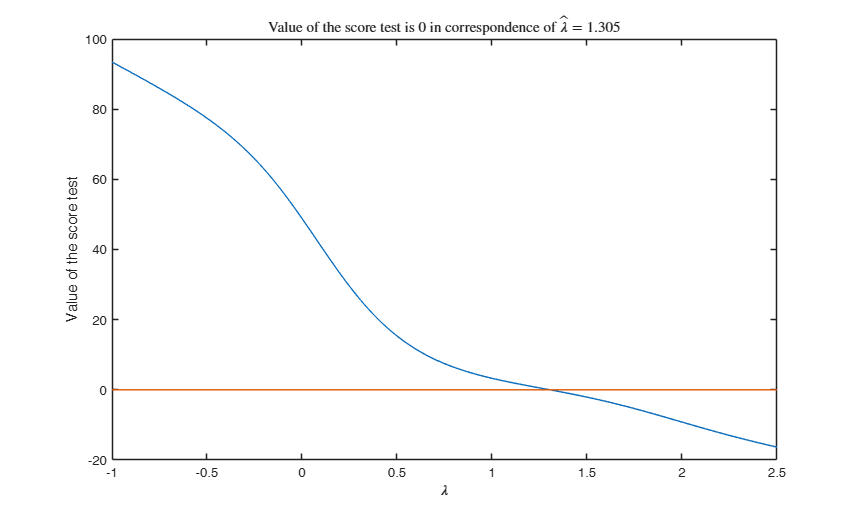# ScoreYJ

Computes the score test for Yeo and Johnson transformation

## Syntax

• outSC=ScoreYJ(y,X)example
• outSC=ScoreYJ(y,X,Name,Value)example

## Description

 outSC =ScoreYJ(y, X) Score with all default options for the wool data.

 outSC =ScoreYJ(y, X, Name, Value) Score with optional arguments.

## Examples

expand all

### Score with all default options for the wool data.

XX=load('wool.txt');
y=XX(:,end);
X=XX(:,1:end-1);
% Score test using the five most common values of lambda
[outSc]=ScoreYJ(y,X);
disp(outSc.Score)

### Score with optional arguments.

Loyalty cards data.

load('loyalty.txt');
y=loyalty(:,4);
X=loyalty(:,1:3);
% la = vector containing the values of the transformation
% parameters which have to be tested
la=[0.25 1/3 0.4 0.5];
[outSc]=ScoreYJ(y,X,'la',la,'intercept',true);

## Related Examples

expand all

### Compare Score test using BoxCox and YeoJohnson for the wool data.

Wool data.

XX=load('wool.txt');
y=XX(:,end);
X=XX(:,1:end-1);
% Define vector of transformation parameters
la=[-1:0.01:1];
% Score test using YeoJohnson transformation
[outYJ]=ScoreYJ(y,X,'la',la);
% Score test using YeoJohnson transformation
[outBC]=Score(y,X,'la',la);
plot(la',[outBC.Score outYJ.Score])
xlabel('\lambda')
ylabel('Value of the score test')
legend({'BoxCox' 'YeoJohnson'})

###Score test using Darwin data given by Yeo and Yohnson.

y=[6.1, -8.4, 1.0, 2.0, 0.7, 2.9, 3.5, 5.1, 1.8, 3.6, 7.0, 3.0, 9.3, 7.5 -6.0]';
n=length(y);
X=ones(n,1);
la=-1:0.01:2.5;
% Given that there are no explanatory variables the test must be
% called with intercept false
out=ScoreYJ(y,X,'intercept',false,'la',la,'Lik',1);
plot(la',out.Score)
xax=axis;
line(xax(1:2),zeros(1,2))
xlabel('\lambda')
ylabel('Value of the score test')
title('Value of the score test is 0 in correspondence of $\hat \lambda =1.305$','Interpreter','Latex')## Input Arguments

### y — Response variable. Vector.

A vector with n elements that contains the response variable. It can be either a row or a column vector.

Data Types: single| double

### X — Predictor variables. Matrix.

Data matrix of explanatory variables (also called 'regressors') of dimension (n x p-1). Rows of X represent observations, and columns represent variables.

Missing values (NaN's) and infinite values (Inf's) are allowed, since observations (rows) with missing or infinite values will automatically be excluded from the computations.

Data Types: single| double

### Name-Value Pair Arguments

Specify optional comma-separated pairs of Name,Value arguments. Name is the argument name and Value is the corresponding value. Name must appear inside single quotes (' '). You can specify several name and value pair arguments in any order as  Name1,Value1,...,NameN,ValueN.

Example:  'intercept',false , 'la',[0 0.5] , 'Lik',false , 'nocheck',true 

### intercept —Indicator for constant term.true (default) | false.

Indicator for the constant term (intercept) in the fit, specified as the comma-separated pair consisting of 'Intercept' and either true to include or false to remove the constant term from the model.

Example:  'intercept',false 

Data Types: boolean

### la —transformation parameter.vector.

It specifies for which values of the transformation parameter it is necessary to compute the score test.

Default value of lambda is la=[-1 -0.5 0 0.5 1]; that is the five most common values of lambda

Example:  'la',[0 0.5] 

Data Types: double

### Lik —likelihood for the augmented model.boolean.

If true the value of the likelihood for the augmented model will be produced else (default) only the value of the score test will be given

Example:  'Lik',false 

Data Types: logical

### nocheck —Check input arguments.boolean.

If nocheck is equal to true no check is performed on matrix y and matrix X. Notice that y and X are left unchanged. In other words the additional column of ones for the intercept is not added. As default nocheck=false.

Example:  'nocheck',true 

Data Types: boolean

## Output Arguments

### outSC — description Structure

Containing the following fields:

Value Description
Score

score test. Scalar. t test for additional constructed variable

Lik

value of the likelihood. Scalar. This output is produced only if input value Lik =1

Yeo, I.K. and Johnson, R. (2000), A new family of power transformations to improve normality or symmetry, "Biometrika", Vol. 87, pp. 954-959.